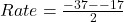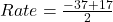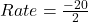## This table shows values that represent a quadratic function. х y 0 -1 1 SON | N|مي | | -10

Question

This table shows values that represent a quadratic function.
х
y
0
-1
1
SON
| N|مي | |
-10
4
-17
-26
6
-37
What is the average rate of change for this quadratic function for the interval
from x= 4 to x= 6?
A. 10
B. -10
C. 20
D. -20

in progress 0
2 weeks 2021-09-05T15:38:20+00:00 1 Answers 0 views 0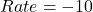Step-by-step explanation:

Given

The table

Required

The average rate if change over (4,6)

This is calculated as: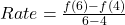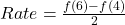From the table: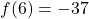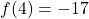So: